Warning

This documents an unmaintained version of NetworkX. Please upgrade to a maintained version and see the current NetworkX documentation.

# line_graph¶

line_graph(G, create_using=None)[source]

Returns the line graph of the graph or digraph G.

The line graph of a graph G has a node for each edge in G and an edge joining those nodes if the two edges in G share a common node. For directed graphs, nodes are adjacent exactly when the edges they represent form a directed path of length two.

The nodes of the line graph are 2-tuples of nodes in the original graph (or 3-tuples for multigraphs, with the key of the edge as the third element).

For information about self-loops and more discussion, see the Notes section below.

Parameters: G (graph) – A NetworkX Graph, DiGraph, MultiGraph, or MultiDigraph. L – The line graph of G. graph

Examples

>>> import networkx as nx
>>> G = nx.star_graph(3)
>>> L = nx.line_graph(G)
>>> print(sorted(map(sorted, L.edges())))  # makes a 3-clique, K3
[[(0, 1), (0, 2)], [(0, 1), (0, 3)], [(0, 2), (0, 3)]]


Notes

Graph, node, and edge data are not propagated to the new graph. For undirected graphs, the nodes in G must be sortable, otherwise the constructed line graph may not be correct.

Self-loops in undirected graphs

For an undirected graph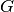without multiple edges, each edge can be written as a set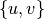. Its line graph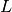has the edges ofas its nodes. If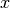and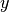are two nodes in, then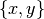is an edge inif and only if the intersection ofandis nonempty. Thus, the set of all edges is determined by the set of all pairwise intersections of edges in.

Trivially, every edge in G would have a nonzero intersection with itself, and so every node inshould have a self-loop. This is not so interesting, and the original context of line graphs was with simple graphs, which had no self-loops or multiple edges. The line graph was also meant to be a simple graph and thus, self-loops inare not part of the standard definition of a line graph. In a pairwise intersection matrix, this is analogous to excluding the diagonal entries from the line graph definition.

Self-loops and multiple edges inadd nodes toin a natural way, and do not require any fundamental changes to the definition. It might be argued that the self-loops we excluded before should now be included. However, the self-loops are still “trivial” in some sense and thus, are usually excluded.

Self-loops in directed graphs

For a directed graphwithout multiple edges, each edge can be written as a tuple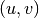. Its line graphhas the edges ofas its nodes. Ifandare two nodes in, then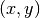is an edge inif and only if the tail ofmatches the head of, for example, if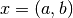and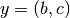for some vertices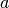,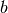, and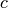in.

Due to the directed nature of the edges, it is no longer the case that every edge inshould have a self-loop in. Now, the only time self-loops arise is if a node initself has a self-loop. So such self-loops are no longer “trivial” but instead, represent essential features of the topology of. For this reason, the historical development of line digraphs is such that self-loops are included. When the graphhas multiple edges, once again only superficial changes are required to the definition.

References

• Harary, Frank, and Norman, Robert Z., “Some properties of line digraphs”, Rend. Circ. Mat. Palermo, II. Ser. 9 (1960), 161–168.
• Hemminger, R. L.; Beineke, L. W. (1978), “Line graphs and line digraphs”, in Beineke, L. W.; Wilson, R. J., Selected Topics in Graph Theory, Academic Press Inc., pp. 271–305.